Next: Capacitors in Series and Up: Capacitance Previous: Capacitance

## Dielectrics

Strictly speaking, the expression (108) for the capacitance of a parallel plate capacitor is only valid if the region between plates is a vacuum. However, this expression turns out to be a pretty good approximation if the region is filled with air. But, what happens if the region between the plates is filled by an insulating material such as glass or plastic?

We could investigate this question experimentally. Suppose that we started with a charged parallel plate capacitor, whose plates were separated by a vacuum gap, and which was disconnected from any battery or other source of charge. We could measure the voltage difference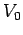between the plates using a voltmeter. Suppose that we inserted a slab of some insulating material (e.g., glass) into the gap between the plates, and then re-measured the voltage difference between the plates. We would find that the new voltage differencewas less than, despite that fact that the chargeon the plates was unchanged. Let us denote the voltage ratio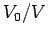as. Since,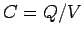, it follows that the capacitance of the capacitor must have increased by a factorwhen the insulating slab was inserted between the plates.

An insulating material which has the effect of increasing the capacitance of a vacuum-filled parallel plate capacitor, when it is inserted between its plates, is called a dielectric material, and the factorby which the capacitance is increased is called the dielectric constant of that material. Of course,varies from material to material. A few sample values are given in Table 1. Note, however, thatis always greater than unity, so filling the gap between the plates of a parallel plate capacitor with a dielectric material always increases the capacitance of the device to some extent. On the other hand,for air is only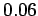percent greater thanfor a vacuum (i.e.,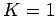), so an air-filled capacitor is virtually indistinguishable from a vacuum-filled capacitor.

Table 1: Dielectric constants of various common materials.
 MaterialVacuum 1 Air 1.00059 Water 80 Paper 3.5 Pyrex 4.5 Teflon 2.1

The formula for the capacitance of a dielectric-filled parallel plate capacitor is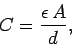(109)

where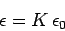(110)

is called the permittivity of the dielectric material between the plates. Note that the permittivity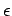of a dielectric material is always greater than the permittivity of a vacuum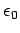How do we explain the reduction in voltage which occurs when we insert a dielectric between the plates of a vacuum-filled parallel plate capacitor? Well, if the voltage difference between the plates is reduced then the electric field between the plates must be reduced by the same factor. In other words, the electric field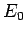generated by the charge stored on the capacitor plates must be partially canceled out by an opposing electric field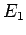generated by the dielectric itself when it is placed in an external electric field. What is the cause of this opposing field? It turns out that the opposing field is produced by the polarization of the constituent molecules of the dielectric when they are placed in an electric field (see Sect. 3.4). Ifis sufficiently small then the degree of polarization of each molecule is proportional to the strength of the polarizing field. It follows that the strength of the opposing fieldis also proportional to. In fact, the constant of proportionality is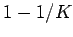, so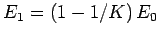. The net electric field between the plates is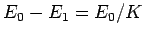. Hence, both the field and voltage between the plates are reduced by a factorwith respect to the vacuum case. In principle, the dielectric constantof a dielectric material can be calculated from the properties of the molecules which make up the material. In practice, this calculation is too difficult to perform, except for very simple molecules. Note that the result that the degree of polarization of a polarizable molecule is proportional to the external electric field-strengthbreaks down ifbecomes too large (just as Hooke's law breaks down if we pull too hard on a spring). Fortunately, however, the field-strengths encountered in conventional laboratory experiments are not generally large enough to invalidate this result.

We have seen that when a dielectric material of dielectric constantis placed in the uniform electric field generated between the plates of a parallel plate capacitor then the material polarizes, giving rise to a reduction of the field-strength between the plates by some factor. Since there is nothing particularly special about the electric field between the plates of a capacitor, we surmise that this result is quite general. Thus, if space is filled with a dielectric medium then Coulomb's law is rewritten as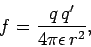(111)

and the formula for the electric field generated by a point charge becomes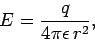(112)

etc. Clearly, in a dielectric medium, the laws of electrostatics take exactly the same form as in a vacuum, except that the permittivity of free spaceis replaced by the permittivity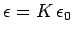of the medium. Dielectric materials have the general effect of reducing the electric fields and potential differences generated by electric charges. Such materials are extremely useful because they inhibit breakdown. For instance, if we fill a parallel plate capacitor with a dielectric material then we effectively increase the amount of charge we can store on the device before breakdown occurs.Next: Capacitors in Series and Up: Capacitance Previous: Capacitance
Richard Fitzpatrick 2007-07-14